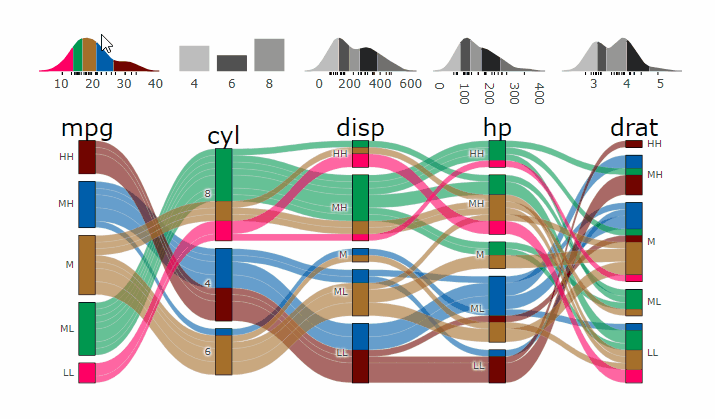`parcats` was released on CRAN. It is an htmlwidget providing bindings to the `plotly.js` parcats trace, which is not supported by the `plotly` R package. Also adds marginal histograms for numerical variables.demogif

# What it can do

I wanted to add interactivity to `easyalluvial` plots for a while now and found that the parcats trace of `plotly.js` would be perfect because brushing with the mouse highlights the entire flow and not just everything flowing in and out of a specific node as in most `D3` Sankey chart implementations. Unfortunately the parcats trace was not available in the `plotly` R package so I decided to build a new html widget to create R bindings for specifically this trace.

• converts any `easyalluvial` plot to an interactive parallel categories diagram
• interactive marginal histograms
• multidimensional partial dependency and model response plots

# easyalluvial

`parcats` requires an alluvial plot created with `easyalluvial` to create an interactive parrallel categories diagram.

# Demo

## Examples

``````suppressPackageStartupMessages( require(tidyverse) )
suppressPackageStartupMessages( require(easyalluvial) )
suppressPackageStartupMessages( require(parcats) )``````

### Parcats from alluvial from data in wide format

``````p = alluvial_wide(mtcars2, max_variables = 5)
parcats(p, marginal_histograms = TRUE, data_input = mtcars2)``````

### Parcats from model response alluvial

Machine Learning models operate in a multidimensional space and their response is hard to visualise. Model response and partial dependency plots attempt to visualise ML models in a two dimensional space. Using alluvial plots or parrallel categories diagrams we can increase the number of dimensions.

Here we see the response of a random forest model if we vary the three variables with the highest importance while keeping all other features at their median/mode value.

``````df = select(mtcars2, -ids )
m = randomForest::randomForest( disp ~ ., df)
imp = m\$importance
dspace = get_data_space(df, imp, degree = 3)
pred = predict(m, newdata = dspace)
p = alluvial_model_response(pred, dspace, imp, degree = 3)
parcats(p, marginal_histograms = TRUE, imp = TRUE, data_input = df)``````